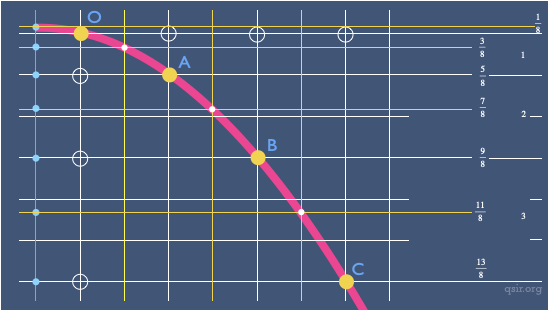## 电学实验的串讲12月17日下午给高三A班上了个大课---7、8两节连上，为了提高课堂效率，提前布置了黑板。小罗同学还记得给上届讲的是图象专题，今年一轮复习还没结束，就选了串讲电学实验的专题...
·[?]电阻测量面面观
·[?]伏安法测电源电动势和内阻
·[?]E、r、R、P
·[?]滑动变阻器的分压与限流

08

## 一道运动学题目的多种解法一物体（不计大小）由静止从斜面顶端下滑到斜面底端，最开始的一段时间下滑了$x_1$，最后一段相同时间下滑了$x_2$，求斜面的总长度$x$

$x_2=a(t-T)T+\frac{1}{2}aT^2$
$x=\frac{1}{2}at^2$

$x_2=v_tT-\frac{1}{2}aT^2$$v_t=\frac{x_1+x_2}{T}$$v_t=2v_\frac{t}{2}$$v_\frac{t}{2}=\frac{\frac{x_1}{T}+\frac{x_2}{T}}{2}$

$(\frac{x_2}{T})^2-(\frac{x_1}{T})^2=2ax'$$x=x'+\frac{x_1+x_2}{2}$

$(\frac{x_2}{T})^2=2ax''$$x=x''+\frac{x_1+x_2}{2}-\frac{x_1}{4}$

$x_1=\frac{1}{2}at^2$ $x_k=\frac{1}{2}a(kt)^2-\frac{1}{2}a((k-1)t)^2$
$\frac{x_k}{x_1}=2k-1$（这里的k只需大于1未必是整数）由此可得
$\frac{x_2}{x_n}=2k-1$得出$k=\frac{x_1+x_2}{2x_1}$
$x=k^2x_1$可得前面结果。P.S.$1=\frac{3}{8}+\frac{5}{8}$$2=\frac{7}{8}+\frac{9}{8}$$3=\frac{11}{8}+\frac{13}{8}$，而最开始的运动时间是$\frac{T}{2}$，对应的位移为$\frac{T}{8}$...
P.S.张子义同学构造平行线构造出初速度为0然后利用13579的方法很巧妙...

去年全国II的答题里，涉及到的数据是$x_1=24m$$x_4=1m$，同上面的分解一样，24=13+11，接下来应该是[9,7],[5,3]，最后的第4秒里只要0.5秒运动，位移为1，倒过来就是[0、1]、[3、5]、[7、9]、[11、13]...

一物体由静止开始以加速度$a_1$匀加速运动了一段时间，加速度突变为$a_2$又经过相同的时间物体恰回到了出发点，求$\frac{a_1}{a_2}$

1、位移为零的标准方法:$\frac{1}{2}aT^2+a_1TT+\frac{1}{2}a_2T^1=0$
2、速度为零的时间t:$\frac{1}{2}a_1T^2+\frac{1}{2}a_2t^2=\frac{1}{2}a_2(T-t)^2$,$a_1T=a_2t$
3、平均速度:$\frac{a_1T}{2}=-\frac{a_1T+a_1T+a_2T}{2}$
4、速度时间图像面积:
5、王上同学设定前后两端时间没的位移和利用平均速度等于中间时刻瞬时速度的方法很巧妙:$v_{\frac{T}{2}}=\frac{x}{T}$,$v_{2T}+2\frac{x}{T}=2\frac{-x}{T}$,$a_1T=2\frac{x}{t}$,$a_2T=-\frac{4x}{T}-\frac{2x}{T}$...
6、张博涵的图像割补法，有学生称之为飞镖的割补法也很通用...

P.S.俊达问了道题目，他好奇有没有“帅”的方法
“匀加速运动的物体，从A到B速度增加了v，位移为$x_1$，从B到D速度增加了2v，位移为$x_2$，C为BD中间时刻经过的点，求BC的位移$x$

Update202103·可能有点用的物理学习资料[?]